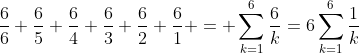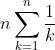## Wednesday, 12 January 2011

### Getting the Full SetI recently wrote about some different forms of probability distributions, Binomial, Multinomial, Geometric and Poisson. Today I want to address a pretty type of problem that doesn't fit any of those.

Everybody who plays around much with recreational mathematics has encountered the famous "birthday problem." It is often stated similar to this: "How many randomly selected people must be assembled so that the probability that at least two of them have the same birthday is at least p (for some specific p, often 1/2)."

But today I want you to think about a slightly different variation. How many people (on average) would you have to gather in order to have at least one with a birthday on every day of the year" (like the birthday problem, we make some assumptions that are not exactly true... births occur with equal probability on every day of the year (they don't, see here. consider only 365 days a year, etc..)

That's probably a hard one to start, so let's break down to an easier problem. How many times should you have to flip a coin until you get at least one head and one tail?

The probability you get one of them on the first roll is 1. But how long should it take to get the "other" one. Well, each flip the probability it happens is p=1/2, and we know that the expected value of this geometric event is Ev= 1/p.. or 2 flips. So we figure that, on average, we will get both outcomes in three flips. To be suggestive, I will suggest that you see that as 2/2 + 2/1.

Ok, upping the ante a little. How many times would you have to roll a die to get all six possible outcomes? Again, break it into packets. How many times should you have to roll until you get one of the six outcomes? Good, you recognize that ONE of them has to happen on the first roll. Now to get one of the five others on the following rolls has a probability of 5/6, so it should take only 6/5 rolls (on average) to get a second outcome. By now you can see we will probably end up with 1+6/5+6/4+...+6/1. I point out here that that can be written as.
Ok, but that summation of 1/k as k goes from one to some number is a harmonic series. It grows very slowly, and no body wants to add up 365 fractions that get very small very quickly. Fortunately, Euler came to our rescue. What Euler did was come up with a really good approximation for 1/1 + 1/2 + 1/3 + ..... + 1/n for any n... It turns out that as n gets bigger and bigger, the sum gets closer and closer to n*ln(n).. and Euler came up with a really good estimate of how wrong it would be. As n gets really big the sum ofis about n ln(n) + .577. So 365 outcomes is not much more difficult to calculate than six was. (365)ln(365)+.577 turns out to be about 2154 people. So if you go to a pretty big school, maybe there is a person enrolled who has a birthday on each day of the year.

So you need a challenge.... Suppose you don't have 2154 people on campus. What if you have say 1000. Now what is the probability that there is someone on campus with all 365 birth dates.

Be the first on YOUR campus to solve it, and send the solution here. It might be easier to start by making the problem smaller.

td said...

Nice blogpost! Thanks!
Are you going to give away the solution to the challenge?

Pat's Blog said...

td, Thanks for the kind words....

Solution??? well I will give a very close approximation to the solution...and write about some different approaches to a solution (None of which are very easy, at least for me) in a (not to far in the) future blog.Anonymous said...

There is a fascinating wording problem with: "Now what is the probability that there is someone on campus with all 365 birth dates."

Jonathan

Fred Gloff said...

So close and yet so far! You were genuinely on the right track in the paragraph beginning with “I reasoned that the probability . . .”, but you skipped one step before using the Poisson distribution. You had correctly computed the probability of a particular birthdate not occurring among the 1000 people. But that applies to each of 365 birthdates. So we should multiply that probability by 365, thus finding that the expected value of the number of birthdates NOT occurring is 23.486…. Notice that we have used an exact technique to obtain this result. If this expected frequency had turned out to be merely 4 or 5, the probability of no missed birthdates would be “pretty low.” When it is larger than 23, that probability must be extremely small. If you want to get a handle on how small, now is the time to reach for the Poisson distribution, or since we are talking about getting zero occurrences, just compute e^(-23.486), getting about 6E-11 (Now we are approximating). Notice that carrying out these computations on a basic scientific calculator would take little more than 10 seconds.
But we are not done yet. Even if one is not familiar with the Poisson distribution, it should seem apparent that the probability distribution for the number of missed birthdays would be skewed to the right (many more options above 23.5 than below), so the mode would be smaller than 23.5. In the Poisson distribution, it is right at 23, with a probability of about 8.3%. So, as Jon Ingram found by the matrix method, the most likely outcome is 342 distinct birthdays. How do our probabilities compare with his? The first is high (but the discrepancy is hardly meaningful) and the second is low. For those approximations, I actually prefer to use a binomial distribution. With “birthday problems”, using an n-value about half the maximum number of possibilities (364/2 here) seems to work fairly well. With n=182, the probabilities come out around 1E-11 and 8.8%, clearly closer to Jon’s results. Sometimes being willing to accept approximate answers can produce useful results while saving a tremendous amount of effort in both reasoning and computation.
In the previous day’s post, Pat showed that the average group size needed to cover all 365 possible birthdates is about 2154. Using that in place of 1000 produces an expected frequency of .990 (pretty close to 1, which is typical when dealing with waiting time random variables) and a probability of about 37% (not far from e^-1). With a group size of 2284, we get an expected frequency of 0.693 (near ln(1/2)), with a probability very close to 50%. Thus the median waiting time is approximately 2284.
Fred

Fred Gloff said...

My recent comment should have included the following:
But we are not done yet. By using the binomial distribution with parameters 1000 and 1/365, we can extend the exploration in my first paragraph to obtain the entire frequency distribution for the single, double, triple, etc. birthdates. The table below includes the first five rows, rounded as shown. The first column gives number of matched birthdates, the second gives the mean number of times a matched group of that size would occur, and the third gives the mean number of people that would fit into that group size.
0 23.5 0.0
1 64.5 64.5
2 88.5 177.1
3 80.9 242.8
4 55.4 221.6
Notice that the most frequent group type is the matched pairs, with matched triples close behind. On the other hand, individuals are most likely to belong to a matched triple, with matched quadruples next in line. I would encourage interested readers to look at a larger portion of the table, using either a spreadsheet or a calculator with good list-handling capabilities. One feature to observe is the sums in the 2nd and 3rd columns. The 2nd column adds to 365 (no surprise since it is 365 times a probability distribution), and the 3rd totals 1000—confirmation that every possible birthdate is counted exactly once, and every person is accounted for exactly once. I first noticed this many years ago when dealing with an analogous situation, using MUCH larger numbers, and still consider it one of many great examples of the beauty of mathematics.
Fred
P.S.: This system will not permit the spacing between columns that I have input. I hope you can interpret the table in spite of this.

Fred Gloff said...

I was so annoyed at the distorted appearance of the table that I decided to try it another way, that should be much more readable. I also changed the 0.0 to just 0, because unlike the other 9 values in the last two columns, it is not the result of rounding off.
0 -----> 23.5 --------> 0
1 -----> 64.5 ------> 64.5
2 -----> 88.5 -----> 177.1
3 -----> 80.9 -----> 242.8
4 -----> 55.4 -----> 221.6
But there is more (Isn’t there always?). Now that we have an easy way of getting the mean number of matched birthday groups (call it M), we can use that value and a normal approximation to the discrete distribution to get a handle on the range of frequencies to expect. Using SQRT(M) as an overestimate of the standard deviation, compute M-2*SQRT(M) and M+2*SQRT(M) and round inward to the next integer to create a +/-2 standard deviation interval, enclosing about 95.5% of the cases. Using this rule of thumb for the case of quadruple birthdates, with M=55.4, the two endpoints are 40.5 and 70.3 (nearest tenth is enough), so about 96% of the time the number of quadruple birthdates would fall in the 41 to 70 interval. Because we have overestimated the SD (more pronounced with large values of M), a shorter interval (43 to 68) is actually sufficient, but for simplicity, I don’t think this is a big concern.
When M is small (say below 7) the normal approximation does not work quite as well, so we need a little adjustment. Let’s use M=5.3 as an example. The computed endpoints are at 0.7 and 9.9, but the interval from 1 to 9 is not quite enough. Because the 9.9 is so close to 10 (within 0.2), we need to include that frequency as well. This is not an issue for the left-hand endpoint.
Don’t use this method on the expected frequencies in the third column. For the case of the number of people that are in a group of 4 matching birthdates (avg. = 221.6), take the interval we previously found and multiply it by 4, getting a 164 to 280 interval. Can you explain why?
Fred